NEET  >  Moment of Inertia

# Moment of Inertia Notes | Study Physics Class 11 - NEET

## Document Description: Moment of Inertia for NEET 2022 is part of Physics Class 11 preparation. The notes and questions for Moment of Inertia have been prepared according to the NEET exam syllabus. Information about Moment of Inertia covers topics like and Moment of Inertia Example, for NEET 2022 Exam. Find important definitions, questions, notes, meanings, examples, exercises and tests below for Moment of Inertia.

Introduction of Moment of Inertia in English is available as part of our Physics Class 11 for NEET & Moment of Inertia in Hindi for Physics Class 11 course. Download more important topics related with notes, lectures and mock test series for NEET Exam by signing up for free. NEET: Moment of Inertia Notes | Study Physics Class 11 - NEET
 1 Crore+ students have signed up on EduRev. Have you?

Linear momentum is a product of the mass (m) of an object and the velocity (v) of the object. If an object has higher momentum, then it harder to stop it. The formula for linear momentum is p = mv. The total amount of momentum never changes, and this property is called conservation of momentum. Let us study more about Linear momentum and conservation of momentum.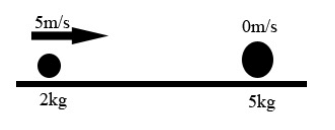Linear Momentum of System of Particles
We know that the linear momentum of the particle is
p = mv
Newton’s second law for a single particle is given by,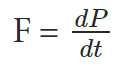where F is the force of the particle. For ‘ n ‘ no. of particles total linear  momentum is,
P = p+ p+…..+pn
each of momentum is written as  mv+ m2v+ ………..+mnvn. We know that velocity of the centre of mass is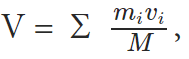mv =  Σ  mivi
So comparing these equations we get,
P = M V
Therefore we can say that the total linear momentum of a system of particles is equal to the product of the total mass of the system and the velocity of its center of mass. Differentiating the above equation we get,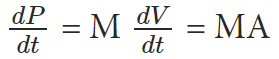dv/dt is acceleration of centre of mass, MA is the force external. So,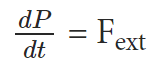This above equation is nothing but Newton’s second law to a system of particles. If the total external force acting on the system is zero,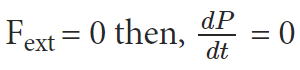This means that P = constant. So whenever the total force acting on the system of a particle is equal to zero then the total linear momentum of the system is constant or conserved. This is nothing but the law of conservation of total linear momentum of a system of particles.

Conservation of Total Linear Momentum of a System of Particles

Let us take the example of radioactive decay. What is radioactive decay? It is a process where an unstable nucleus splits up in relatively stable nuclei releasing a huge amount of energy.
Suppose there is a parent nucleus which is unstable and it wants to become stable, in order to attain stability it will emit α particle and another daughter nucleus.
This daughter nucleus is much more stable than the parent nucleus. This what radioactive decay is. Now suppose the parent nucleus is at rest and also the mass of the α is ‘ m ‘ and the daughter nucleus is M.
So the mass of the parent nucleus will be m + M. Here everything that is happening is not due to the external force but all that happens is due to the internal force. So here Fext = 0, we can say that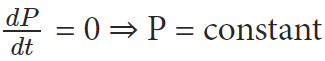The document Moment of Inertia Notes | Study Physics Class 11 - NEET is a part of the NEET Course Physics Class 11.
All you need of NEET at this link: NEET

## Physics Class 11

126 videos|449 docs|210 tests
 Use Code STAYHOME200 and get INR 200 additional OFF

## Physics Class 11

126 videos|449 docs|210 tests

### How to Prepare for NEET

Read our guide to prepare for NEET which is created by Toppers & the best Teachers

Track your progress, build streaks, highlight & save important lessons and more!

,

,

,

,

,

,

,

,

,

,

,

,

,

,

,

,

,

,

,

,

,

;